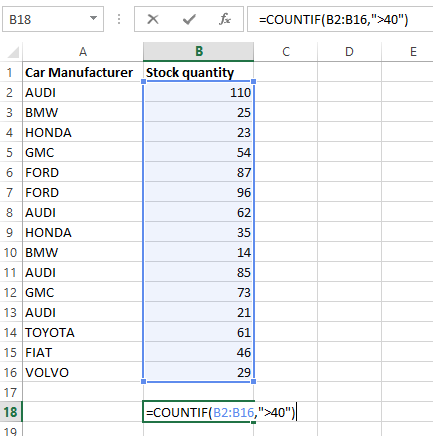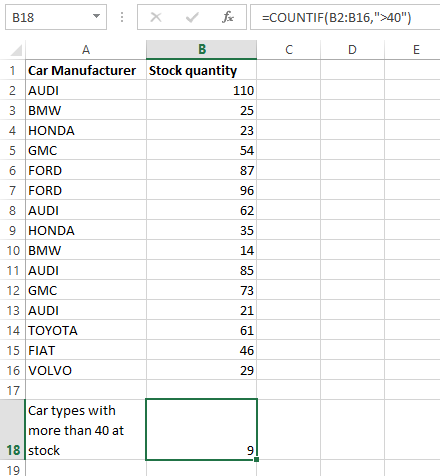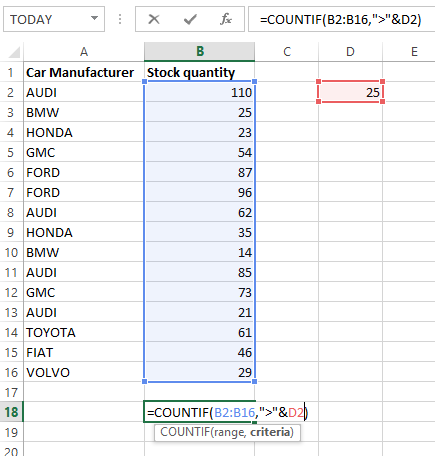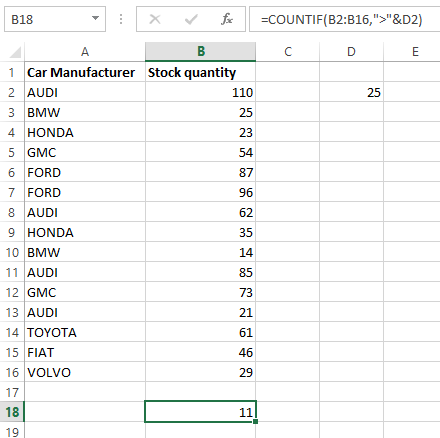# How to use COUNTIF greater thanCOUNTIF() function is used for counting cells with unique values in a range that meet a certain criterion, or condition. This can be used for any type of data including text, numbers, dates or even blank cells.

The syntax of the COUNTIF() function is very simple when compared to some other excel functions.

COUNTIF(range, criteria)

It has two arguments where range describes the range of cell/s to count and criteria explains the condition or criterion for counting.

Greater than logic can be applied to certain practical uses, particularly for numerical and date formats.

To count values greater than the value you specify, you have to simply add the greater than “>” operator to the criteria. See the sample syntax below.

=COUNTIF(A2:A20,">20")

Note that the operator (i.e. “>”) and the conditional value should always be enclosed in double quotations.

Example 1

The following example illustrate how to count items which has cell value more than a certain number.The range of cells have been selected as B2:B16 and the condition is “greater than 40”.If you want to count items which are greater than or equal to certain value then use the corresponding operator (i.e. “>=”).

It is also possible to use the above function to count cells based on another cell’s value. For this you have to first enclose the operator in double quotes (“>”) and then add an ampersand (&) before the cell value to be included. See the following syntax for better understanding.

=COUNTIF(A2:A20,”>”&B2)

Which will count cells in the range of A2:A20 where the cell value is greater than B2. When using a cell value as the criteria, it does not need to be enclosed in double quotes.Note: This function can also be used with date format. See the following sample syntaxes:

=COUNTIF(D3:D19,">6/4/2016")             count cells which have dates greater than 6/4/2016

=COUNTIF(A2:A20,">"&TODAY())         count cells which have dates greater than today